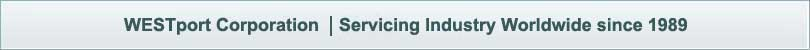# Correcting Measurement Biases

For Rockwell hardness machines that have successfully passed both direct and indirect verifications, there will continue to be some level of measurement error or bias with respect to the reference standards. If this bias is felt to be significant for the user’s application, then it may be advantageous to make mathematical corrections based on the certified values of the reference test blocks. Presently, ASTM and ISO test methods do not address making mathematical corrections, although, in practice, a form of mathematical correction is commonly made for newer Rockwell machines capable of being electronically calibrated. These machines can determine correction curves by comparing certified test block values with values measured during an indirect verification. The curves are electronically stored in the Rockwell machine and correct future measurement values based of this curve. In general, mathematical corrections should only be made in cases where the Rockwell machine has been successfully verified.

A practical method for applying corrections for the measurement biases is to determine a linear calibration curve for the entire hardness scale. The linear calibration curve provides a correction value to be applied to future hardness measurements at any hardness level of that scale. The correction value is dependent on the Rockwell scale and hardness level of the material under test. A linear correction curve is chosen because indirect verifications are usually made with test blocks at only three levels of hardness for each Rockwell scale A curvilinear fit to only three data points often can produce impractical results at hardness levels other than the three verified levels. A separate and distinct correction curve is required for each Rockwell scale.

Figure 9 graphically illustrates how the biases can be corrected for a single Rockwell scale. The left axis represents the difference between testing machine measurements made on reference test block standards and the certified values of the reference test blocks. This is the error or bias determined as part of an indirect verification (average of machine measurements minus certified reference value). The bottom axis represents the hardness level that is measured. In this example, the round points indicate the bias values determined by testing three reference test block standards. A linear fit is made to the three bias values. The correction to be applied to future measurements is determined from the value at each point along the linear fit line. For example, when a future measurement is made at 25 HRC, the correction would be to add approximately 0.25 HRC to the measured value. The square data points and dashed line illustrate the result of applying these corrections to the bias values.